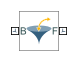# Inverse Square Law Force

Force proportional to the inverse square distance between two frame origins

## Library

Forces and Torques

•## Description

This block represents a force pair that is inversely proportional to the square distance between the base and follower frame origins. The two forces in the pair have equal magnitude but opposite directions. One force acts on the base frame origin, along the vector connecting the follower to base frame origins. The other force acts on the follower frame origin, along the vector connecting base to follower frame origins.

The value of the force depends on a force constant that you specify. A positive force constant represents a repulsive force that pushes the two frame origins apart. A negative force constant represents an attractive force that pulls the two frame origins together.

## Parameters

Force Constant

Specify the proportionality constant of the inverse square law force. This constant is a lumped parameter that encodes the dependence of the force magnitude on the inverse square distance between the two frame origins. The default value is `1`. Select or specify a physical unit.

Sense Force

Select the check box to sense the signed magnitude of the inverse square law force acting between the two frame origins. The block exposes an additional physical signal port to output the force signal. The output signal is a scalar value. This value is positive if the force is repulsive; it is negative if the force is attractive.

## Ports

The block contains frame ports B and F, representing base and follower frames, respectively.

Selecting Sense Force in the block dialog box exposes an additional physical signal port, fm.

## Version History

Introduced in R2012a